# High-power shunt regulator uses BJT & reference IC

Some voltage references can be used as shunt regulators, either by tying VIN and VOUT together or by leaving VIN open. These devices are limited to low currents, however, with a typical limit of around 10 mA. Standard Zener diodes serve the same function, and can operate up to about a watt, but they suffer from high series resistance.

The AD584 voltage reference, along with an external pass transistor, such as the NTE244, allows the synthesis of shunt regulators that can handle as much as 50 W. The circuit shown in Figure 1 can be used to clip high-current, long duration, over-voltage pulses – or it can be used as a floating series voltage drop.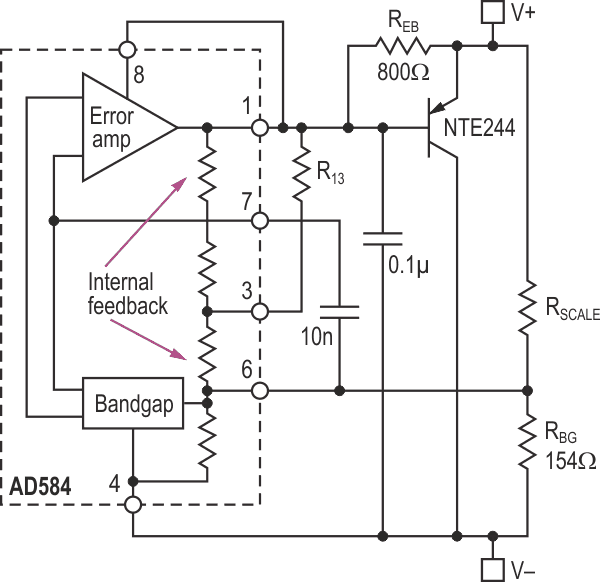Figure 1. High-power shunt regulator.

The AD584 voltage reference combines a precise band-gap reference cell, an error amplifier, and a feedback network. Direct access to the band-gap’s input allows the internal feedback network to be overridden via the external pass transistor and feedback network. The result is a stable voltage between the V+ and V terminals. R13 and REB are included to properly bias the AD584. The 10 nF and 0.1 µF bypass capacitors are included for stability.

RSCALE can be calculated from: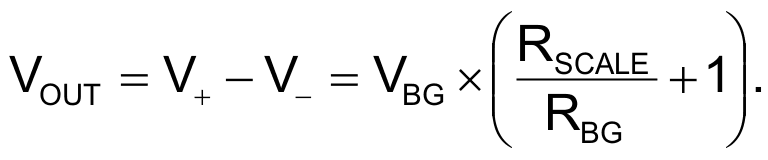where VBG = 1.22 V.

From the data sheet, the internal feedback resistors in the AD584 are 36 kΩ and 12 kΩ, and the desired voltage on pin 3 is V + 2.5 V. Thus, R13 can be calculated from: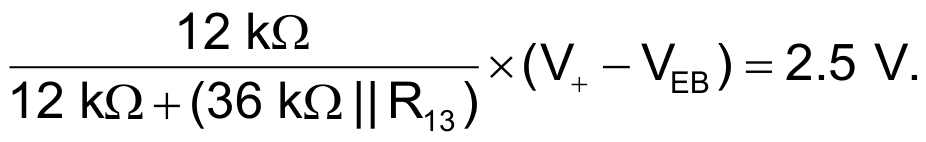This equation simplifies as: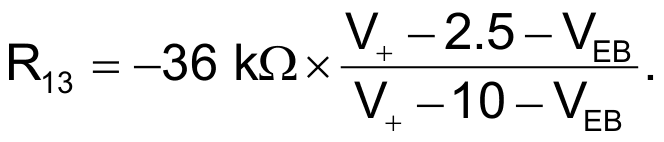For the NTE244, VEB = 1.5 V, so the equation becomes: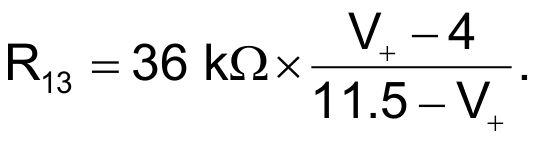The graph in Figure 2 shows the results at five example voltages. The circuit has no problem sinking 5 A at lower voltages, or handling 50 W to 60 W at higher output voltages, subject of course to proper heat sinking and temperature derating of the NTE244 power device. Note that the circuit regulates far better than a Zener diode while operating over a much higher current range.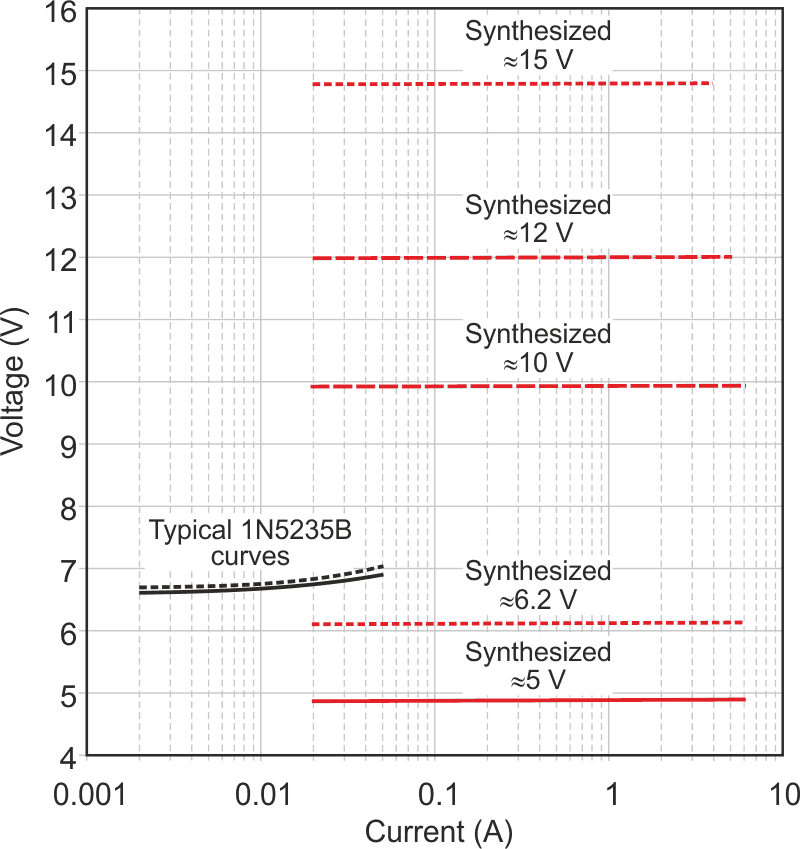Figure 2. Zener diode vs, High Current Synthesized Shunt Regulator.

EDN